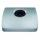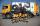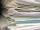# Copper parts

From copper cast were made 3 parts. At first consumed one third of casting, the second 2/3 of rest and the third weighed 18 kg. What is the weight of original copper cast?

Result

x =  81 kg

#### Solution:

x/3+2/3*(x-x/3)+18=x

0.666667x = 54

x = 81

Calculated by our simple equation calculator.

Leave us a comment of example and its solution (i.e. if it is still somewhat unclear...):

Showing 0 comments:Be the first to comment!#### To solve this verbal math problem are needed these knowledge from mathematics:

Need help calculate sum, simplify or multiply fractions? Try our fraction calculator. Do you have a system of equations and looking for calculator system of linear equations?

## Next similar examples:

1. Three granariesThree granaries hold 7,800 metric cent (q) of grain. In the first, 70 tonnes of grain was more than the second and in the third it was 120 tonnes less than in the second. How many grain were in each granary?
2. TreesAlong the road were planted 250 trees of two types. Cherry for 60 CZK apiece and apple 50 CZK apiece. The entire plantation cost 12,800 CZK. How many was cherries and apples?
3. Hotel roomsIn the 45 rooms, there were 169 guests, some rooms were three-bedrooms and some five-bedrooms. How many rooms were?
4. TheatroTheatrical performance was attended by 480 spectators. Women were in the audience 40 more than men and children 60 less than half of adult spectators. How many men, women and children attended a theater performance?
5. Two numbersWe have two numbers. Their sum is 140. One-fifth of the first number is equal to half the second number. Determine those unknown numbers.
6. Two numbersThe sum of the two numbers is 1. Find both numbers if you know that half of the first is equal to one seventh of the second number.
7. Barrel 4Barrel of water weighs 63 kg. After off 75% water, the weight of the barrel with water is 21 kg. How many kg weigh empty barrel and how many kgs water in it?
8. Collecting dayAt collecting day at school pupils of three classes took total of 1063 kg of paper. Class B collected half more than A Class and class C 55 kg more than B. How many kg of paper collected each class?
9. Three brothersThe three brothers have a total of 42 years. Jan is five years younger than Peter and Peter is 2 years younger than Michael. How many years has each of them?
10. Linear systemSolve this linear system (two linear equations with two unknowns): x+y =36 19x+22y=720
11. Equations - simpleSolve system of linear equations: x-2y=6 3x+2y=4
12. EquationsSolve following system of equations: 6(x+7)+4(y-5)=12 2(x+y)-3(-2x+4y)=-44
13. MushroomsEva and Jane collected 114 mushrooms together. Eve found twice as much as Jane. How many mushrooms found each of them?
14. Father and sonFather and son weigh together 108kg. The father weighs 2.6 times more than his son. How much does weight my father and son count?
15. Value 4If 5/18=425 what is the value 13/18?
16. Iron collectingClass 7A collected 3.2 tonnes of iron more than class 7B. Together they collected 6.4 tonnes of iron to the secondary raw material collection. How much did each class collect?
17. PC disksPeter has 45 disks in three colors. One-fifth of the disks are blue, red are twice more than the white. How much is blue, red and white disks?